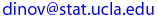## One- and Two-Way Analysis of Variance Java AppletUCLA Statistics

The SOCR 1-Way ANOVA Applet is available here and 2-Way ANOVA is available here (both require Java-enabled browser). fter clinking this link, if you do not see it in the next 5 seconds it pronbably means your browser is NOT Java-enabled!

Analysis of variance (ANOVA) performs comparisons like the t-Test, but for an arbitrary number of factors. Each factor can have an arbitrary number of levels. Furthermore each factor combination can have any number of replicates. ANOVA works on a single dependent variable. The factors must be discrete. The ANOVA can be thought of in a practical sense as an extension of the t-Test to an arbitrary number of factors and levels. It can also be thought of as a linear regression model whose independent variables are restricted to a discrete set.

Last modified on by.

Ivo D. Dinov, Ph.D., Departments of Statistics and Neurology, UCLA School of Medicine